### IMO Shortlist 1961 problem 6

Kvaliteta:
Avg: 0,0
Težina:
Avg: 0,0
Consider a plane$\epsilon$ and three non-collinear points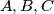$A,B,C$ on the same side of$\epsilon$; suppose the plane determined by these three points is not parallel to$\epsilon$. In plane$\epsilon$ take three arbitrary points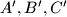$A',B',C'$. Let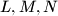$L,M,N$ be the midpoints of segments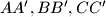$AA', BB', CC'$; Let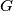$G$ be the centroid of the triangle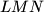$LMN$. (We will not consider positions of the points$A', B', C'$ such that the points$L,M,N$ do not form a triangle.) What is the locus of point$G$ as$A', B', C'$ range independently over the plane$\epsilon$?
Izvor: Međunarodna matematička olimpijada, shortlist 1961